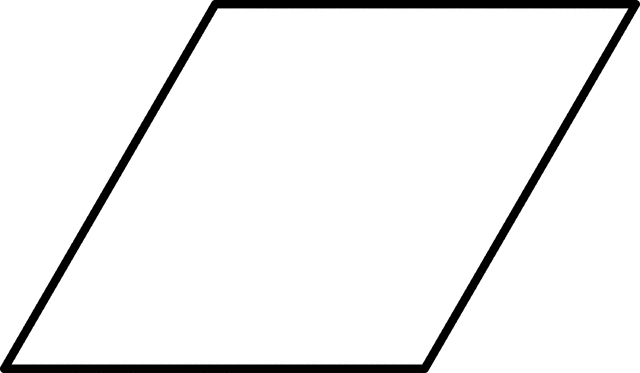GeeksforGeeks App
Open AppBrowser
Continue

# Program for Circumference of a Parallelogram

Given the sides of Parallelogram then calculate the circumference.
Examples :

```Input: a = 10, b = 8
Output: 36.00

Input: a = 25.12, b = 20.4
Output: 91.04```

The opposite sides of a parallelogram are of equal length and parallel. The angles are pairwise equal but not necessarily 90 degree. The circumference of a Parallelogram can be computed as sum of two adjacent sides each multiplied by 2.Formula used to calculate circumference of a Parallelogram:
(2*a)+(2*b)

## C++

 `// C Program to calculate the``// Circumference of a Parallelogram.``#include `` ` `float` `circumferenceparallelogram(``float` `a, ``float` `b)``{``    ``return` `((2 * a) + (2 * b));``}`` ` `int` `main()``{``    ``float` `a = 10, b = 8;``    ``printf``(``"Circumference of a given Parallelogram is : %.2f"``,``           ``circumferenceparallelogram(a, b));``    ``return` `0;``}`

## Java

 `// Java Program To Calculate``// Circumference of a Parallelogram``import` `java.io.*;`` ` `class` `GFG``{``    ``static` `float` `circumferenceparallelogram(``float` `a, ``float` `b)``    ``{``    ``return` `((``2` `* a) + (``2` `* b));``    ``}`` ` `    ``// Driver code``    ``public` `static` `void` `main(String arg[])``    ``{``        ``float` `a = ``10``, b = ``8``;``        ``System.out.print(``"Circumference of a given Parallelogram is :"``);``        ``System.out.println(circumferenceparallelogram(a, b));``    ``}``}`` ` `// This code is contributed by Anant Agarwal.`

## Python3

 `# Python3 Program to calculate the``# Circumference of a Parallelogram`` ` `# Utility Function``def` `circumferenceparallelogram(a,b):``    ``return` `((``2` `*` `a) ``+` `(``2` `*` `b))`` ` `# Driver Function``a ``=` `10``b ``=` `8`` ` `print``(``"Circumference of a given Parallelogram is :"``,``         ``round``(circumferenceparallelogram(a, b),``4``))`` ` `# This code is contributed``# by Azkia Anam.`

## C#

 `// C# Program To Calculate``// Circumference of a Parallelogram``using` `System;`` ` `class` `GFG``{``    ``static` `float` `circumferenceparallelogram(``float` `a,``                                            ``float` `b)``    ``{``    ``return` `((2 * a) + (2 * b));``    ``}`` ` `    ``// Driver code``    ``public` `static` `void` `Main()``    ``{``        ``float` `a = 10, b = 8;``        ``Console.Write(``"Circumference of a"` `+ ``                       ``"given Parallelogram is :"``);``                        ` `        ``Console.Write(circumferenceparallelogram(a, b));``    ``}``}`` ` `// This code is contributed by vt_m.`

## PHP

 ``

## Javascript

 ``

Output :

`Circumference of a given parallelogram is : 36.0`

Time Complexity: O(1)

Auxiliary Space: O(1)

My Personal Notes arrow_drop_up